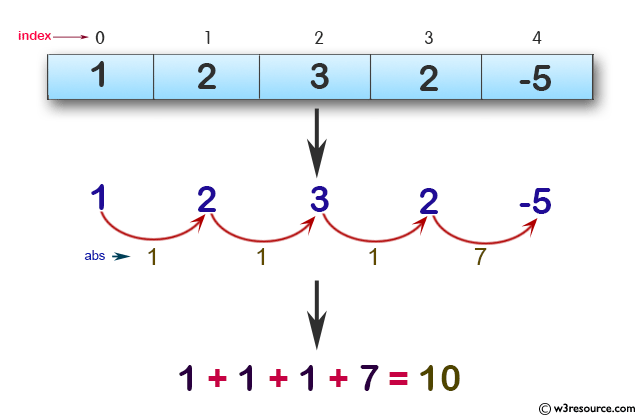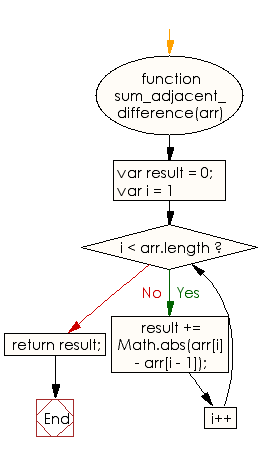# JavaScript: Compute the sum of absolute differences of consecutive numbers of a given array of integers

## JavaScript Basic: Exercise-96 with Solution

Write a JavaScript program to compute the sum of absolute differences of consecutive numbers of a given array of integers.

Pictorial Presentation:Sample Solution:

HTML Code:

``````<!DOCTYPE html>
<html>
<meta charset="utf-8">
<meta name="viewport" content="width=device-width">
<title> Compute the sum of absolute differences of consecutive numbers of a given array of integers</title>
<body>

</body>
</html>
```
```

JavaScript Code:

``````function sum_adjacent_difference(arr) {
var result = 0;
for (var i = 1; i < arr.length; i++) {
result += Math.abs(arr[i] - arr[i - 1]);
}
return result;
}

```
```

Sample Output:

```10
```

Flowchart:ES6 Version:

``````function sum_adjacent_difference(arr) {
let result = 0;
for (let i = 1; i < arr.length; i++) {
result += Math.abs(arr[i] - arr[i - 1]);
}
return result;
}

``````

Live Demo:

See the Pen javascript-basic-exercise-96 by w3resource (@w3resource) on CodePen.

What is the difficulty level of this exercise?

Test your Programming skills with w3resource's quiz.

﻿

## JavaScript: Tips of the Day

Checks if a string is an anagram of another string (case-insensitive, ignores spaces, punctuation and special characters)

Example:

```const isAnagram = (str1, str2) => {
const normalize = str =>
str
.toLowerCase()
.replace(/[^a-z0-9]/gi, '')
.split('')
.sort()
.join('');
return normalize(str1) === normalize(str2);
};
console.log(isAnagram('iceman', 'cinema')); // true
```

Output:

```true
```